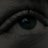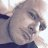# Multi-TimeFrame Stochastic Momentum Index for ThinkorSwim

T

#### theLEMband

##### New member
VIP
Here is a multiple timeframe modification of TOS' built in Stochastic Momentum Index.Code:
``````#multiple timeframe stochastic momentum

declare lower;

input tf = {"MIN", "TWO_MIN", default "FIVE_MIN", "FIFTEEN_MIN", "HOUR", "TWO_HOURS", "FOUR_HOURS", "DAY", "WEEK", "MONTH"};

def timeframe;

switch (tf){
case "MIN":
timeframe = AggregationPeriod.MIN;
case "TWO_MIN":
timeframe = AggregationPeriod.TWO_MIN;
case "FIVE_MIN":
timeframe = AggregationPeriod.FIVE_MIN;
case "FIFTEEN_MIN":
timeframe = AggregationPeriod.FIFTEEN_MIN;
case "HOUR":
timeframe = AggregationPeriod.HOUR;
case "TWO_HOURS":
timeframe = AggregationPeriod.TWO_HOURS;
case "FOUR_HOURS":
timeframe = AggregationPeriod.FOUR_HOURS;
case "DAY":
timeframe = AggregationPeriod.DAY;
case "WEEK":
timeframe = AggregationPeriod.WEEK;
case "MONTH":
timeframe = AggregationPeriod.MONTH;
}

input over_bought = 40.0;
input over_sold = -40.0;
input percentDLength = 4;
input percentKLength = 6;

def min_low = Lowest(low(period = timeframe), percentKLength);
def max_high = Highest(high(period = timeframe), percentKLength);
def rel_diff = close(period = timeframe) - (max_high + min_low) / 2;
def diff = max_high - min_low;

def avgrel = ExpAverage(ExpAverage(rel_diff, percentDLength), percentDLength);
def avgdiff = ExpAverage(ExpAverage(diff, percentDLength), percentDLength);

plot SMI = if avgdiff != 0 then avgrel / (avgdiff / 2) * 100 else 0;
SMI.SetDefaultColor(GetColor(4));

plot AvgSMI = ExpAverage(SMI, percentDLength);
AvgSMI.SetDefaultColor(GetColor(6));

plot overbought = over_bought;
overbought.SetDefaultColor(GetColor(5));
overbought.SetStyle(Curve.SHORT_DASH);

plot oversold = over_sold;
oversold.SetDefaultColor(GetColor(5));
oversold.SetStyle(Curve.SHORT_DASH);``````

Last edited:
T

#### theLEMband

##### New member
VIPAdd the standard TOS SMI, then add this (multiple times if you like) and set them for higher time frame(s). Note that the percent signs on the right are not part of the studies.

Possible use, when higher time frame crosses yellow above green and is above 0, possible buy could occur at next cross of shorter time frame blue above red. Possible sell when higher time frame crosses the other way. Or could hold longer and wait for shorter time frame to cross below -40. For example, right now, 8/15/20, would be looking for next 1 hour blue above red cross (and with yellow still above 0) for possible long entry.

•Katsu and BenTen
K

VIP

T

#### theLEMband

##### New member
VIP
Updated the code in the first post.

K

#### Katsu

##### New member
VIPQQE MTF (Multi Timeframe) for ThinkorSwim Indicators 11How do I use multi timeframe in a watchlist column or scan? Questions 2